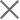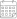Subscribe

### Indexes

July 2022 | Electricity Market Review05 September 2022

In July 2022, Georgian power plants generated 1,308 mln. kWh of electricity (Figure 1). This represents a 5% increase in the total generation compared to the previous year (in July 2021, the total generation was 1,240 mln. kWh). The increase in the generation on a yearly basis comes from a rise of 6% in hydropower generation, and 23% in wind power generation, respectively, more than offsetting a 31% decline in thermal power generation.

On a monthly basis, the generation decreased by approximately 12% (in June 2022, the total generation was 1,485 mln. kWh) (Figure 1). The monthly decrease in the total generation is induced by a 12% decrease in hydropower generation, and a 15% reduction in thermal power generation, while there was a 34% increase in wind power generation.

The consumption of electricity on the local market was 1,147 mln. kWh (-1% compared to July 2021, and +6% compared to June 2022) (Figure 1). In July 2022, power generation exceeded consumption by 161 mln. kWh which was 12% of the total generation and 14% of the total consumption (in July 2021, the difference between the total generation and the consumption resulted in a surplus of 79 mln. kWh, around 6% of the total generation and 7% of the total consumption for the month).